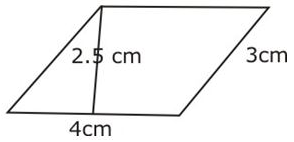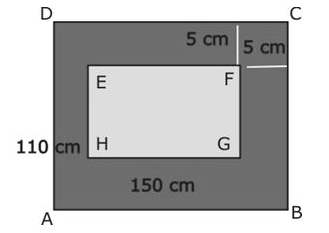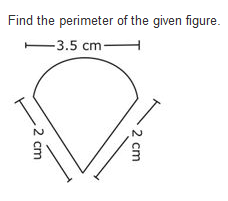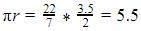Courses

# Test: Mensuration- 3

## 20 Questions MCQ Test Mathematics (Maths) Class 8 | Test: Mensuration- 3

Description
This mock test of Test: Mensuration- 3 for Class 8 helps you for every Class 8 entrance exam. This contains 20 Multiple Choice Questions for Class 8 Test: Mensuration- 3 (mcq) to study with solutions a complete question bank. The solved questions answers in this Test: Mensuration- 3 quiz give you a good mix of easy questions and tough questions. Class 8 students definitely take this Test: Mensuration- 3 exercise for a better result in the exam. You can find other Test: Mensuration- 3 extra questions, long questions & short questions for Class 8 on EduRev as well by searching above.
QUESTION: 1

### The parallel sides of a trapezium are 25 cm and 13 cm. Its non-parallel sides are equal, each being 10 cm. Find the area of the trapezium.

Solution:

Area of trapezium= 1/2*d*(a+b) sq. Units.

Where d= perpendicular distance between parellel sides which are a & b respectively, 25 &13 cm.

25–13=12, 12/2= 6 cm is base of RT. Angled ∆.

Non parallel sides=10cm, this is hypotenuese of this ∆.

Find ‘d ‘ to solve.

d=√{102–62}=8 cm.

Area of trapezium= 1/2*8*(25+13)=152 cm2

QUESTION: 2

Solution:
QUESTION: 3

### Find the altitude of a trapezium, the sum of the lengths of whose bases is 6.5 cm and whose area is 26 cm2.

Solution:
QUESTION: 4

Find the total surface area of a cube whose volume is 343 cm3.

Solution:
volume=(side)^3
so, 343=(side)^3
cube root of 343=side
cube root of 343=7
side=7cm
Surface area=6(side)^2
Surface area=6 x 7^2
Surface area=6 x 49
Surface area=294 cm^2
QUESTION: 5

A cylindrical tank has a capacity of 5632 m3. If the diameter of its base is 16 m, find its depth.

Solution:
QUESTION: 6

Find the area of a rhombus whose diagonals are of lengths 20 cm and 16 cm.

Solution:
QUESTION: 7

Find the area of the given figure.Solution:
QUESTION: 8

Surface area of a cuboid = __________

Solution:
QUESTION: 9

Find the volume of a cuboid whose length is 8 cm, breadth 6 cm and height 3.5 cm.

Solution:

Length of the cuboid = 8 cm

Breadth of the cuboid = 6 cm

Height of the cuboid = 3.5 cm

Volume of the cuboid = length × breadth × height

= 8 x 6 x 3.5 = 168cm3

Therefore,volume of  the cuboid = 168cm3

QUESTION: 10

A godown is in the form of a cuboid of measures 60 m × 40 m × 30 m. How many cuboidal boxes can be stored in it if the volume of one box is 0.8 m3?

Solution:
QUESTION: 11

The perimeter of a trapezium is 52 cm. Its non-parallel sides are 10 cm each and the distance between two parallel sides is 8 cm. Find the area of the trapezium.

Solution:

Perimeter of a Trapezium=sum of all sides
52= sum of parallel sides +20
Sum of parallel sides = 32
Area of Trapezium=
1/2(Sum of parallel sides) x h
=1/2(32)*8 = 128cm2

QUESTION: 12

The cost of papering the wall of a room, 12 m long, at the rate of Rs. 1.35 per square meter is Rs. 340.20. The cost of matting the floor at Re. 0.85 per square metre is Rs. 91.80. Find the height of the room.

Solution:
Let the length of room = l = 12 m
breadth of room = b m
height of room = h m
Cost of matting the floor @ Re. 0.85 per m^2 = Rs. 91.80
floor area = 91.80 / 0.85 = 108 m^2
⇒ l * b = 108
⇒ 12 * b = 108
⇒ b = 108/12
⇒ b = 9 m
cost of papering wall @ Rs. 1.35 per m^2 = Rs. 340.20
wall area is the lateral surface area.
wall area = lateral surface area = 340.20 / 1.35 = 252 m^2
⇒ 2h(l+b) = 252
⇒ 2h(12+9) = 252
⇒ 2h*21 = 252
⇒ h = 252/(2*21)
⇒ h = 6 m
Height of room is 6m.
QUESTION: 13

The area of a trapezium is 384 cm2. Its parallel sides are in the ratio 3:5 and the distance between them is 12 cm. Find the length of each parallel side.

Solution:
QUESTION: 14

In the given figure find the area of the path.Solution:

Area of Rectangle ABCD = 110*150 = 16500cm2
Area of rectangle EFGH = (150-10)(110-10) = 14000cm2
Area of path = 16500-14000 = 2500cm2

QUESTION: 15

The area of a rhombus is 28 cm2 and one of its diagonals is 4 cm. Find its perimeter.

Solution:
QUESTION: 16

Find the side of a cube whose surface area is 2400 cm2.

Solution:
QUESTION: 17

Find the height of a cuboid whose volume is 275 cm3 and base area is 25 cm2

Solution:

Area of the base = Product of length and breadth

Volume of cuboid = Length x breadth x Height
= Area of its base x Height
275 = 25 x Height
Height = 275 / 25 cm
=11 cm

QUESTION: 18

Find the height of cuboid whose volume is 490 cm3 and base area is 35 cm3

Solution:

Volume of cuboid = base area × height
490 = 35 × height
height = 14cm.

QUESTION: 19

Surface area of a cube = _________

Solution:
QUESTION: 20Solution:

Circumference of semi circle =perimeter = sum of two sides + circumference = 4 + 5.5 = 9.5cm